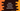# Extension function in Kotlin explanation with examples## Introduction :

Extension functions are used to add more functionality to a Kotlin class easily without inherit from the class. Extension function is a powerful and useful function in Kotlin. For example, we can’t modify any system class or library class, but by using extension function, we can add methods to these classes and we can call them as if they are the actual methods of these classes.

### Example of extension function with user defined class :

We can define one extension function for a user defined class. For example :

``````data class Marks(val subA : Int,val subB: Int,val subC: Int)

fun Marks.getTotal() : Int = this.subA + this.subB + this.subC

fun main(args: Array<String>) {
val m = Marks(40,50,60)
print(m.getTotal())
}``````

Marks class is a data class that takes three integer values as arguments. We have created one extension function for that class getTotal to return the sum of all these three variables. As you have seen in the main function, getTotal() is called as like a normal function defined in that class.

### Example of extension function with system class :

Let’s consider the below example :

``````fun String.removeNonAlphaNumeric() : String = this.filter{ it.isLetterOrDigit() }

fun main() {
var givenString = "Hello-World-!!123"
print(givenString.removeNonAlphaNumeric())
}``````

Here, we have defined removeNonAlphaNumeric as an extension function to the String class. It is used to remove all non alpha numeric characters from a string. Since this is an extension function of String, we can call it with any string variables. this in an extension function is used to access the current caller object.

It will print the below output :

``HelloWorld123``

### Example of extension function with Generic class :

We can use extension function with generic classes in a similar way. For example :

``````fun <T> MutableList<T>.getMiddle(): T = this[(this.size - 1) / 2]

fun main() {
val intList = mutableListOf(1, 2, 3, 4, 5, 6)
val strList = mutableListOf("one", "two", "three")

println(intList.getMiddle())
println(strList.getMiddle())
}``````

Here, we have defined one getMiddle() extension function to return the middle element from a mutable list. It will always return the middle element irrespective of the type of the list.

### Extension functions are dispatched statically :

Extension functions are determined by the type of the expression on which they are called. Let’s consider the below example :

``````open class Marks(val subA: Int, val subB: Int, val subC: Int)
open class ExMarks(val sub1: Int, val sub2: Int, val sub3: Int, val sub4: Int) : Marks(sub1, sub2, sub3)

fun Marks.getTotal(): Int = this.subA + this.subB + this.subC
fun ExMarks.getTotal(): Int = this.sub1 + this.sub2 + this.sub3 + this.sub4

fun findTotal(marks: Marks) {
print(marks.getTotal())
}

fun main(args: Array<String>) {
val m = ExMarks(10, 20, 30, 40)
findTotal(m)
}``````

Here, ExMarks extends Marks. In the main method, we are calling findTotal function with a ExMarks variable that takes one argument of type Marks. It will print 60 because the function is called on typpe Marks, not on ExMarks.

### Member function with same name as extension function :

If we have one member function and one extension function with same name, and same arguments, the member function is preferred.

``````open class Marks(val subA: Int, val subB: Int, val subC: Int){
fun getTotal() = this.subA + this.subB;
}

fun Marks.getTotal(): Int = this.subA + this.subB + this.subC

fun main(args: Array<String>) {
val m = Marks(10, 20, 30)
print(m.getTotal())
}``````

Here, getTotal of Marks class is called, not the extension function. But we can overload one member function with different parameters.